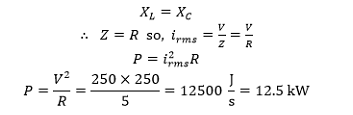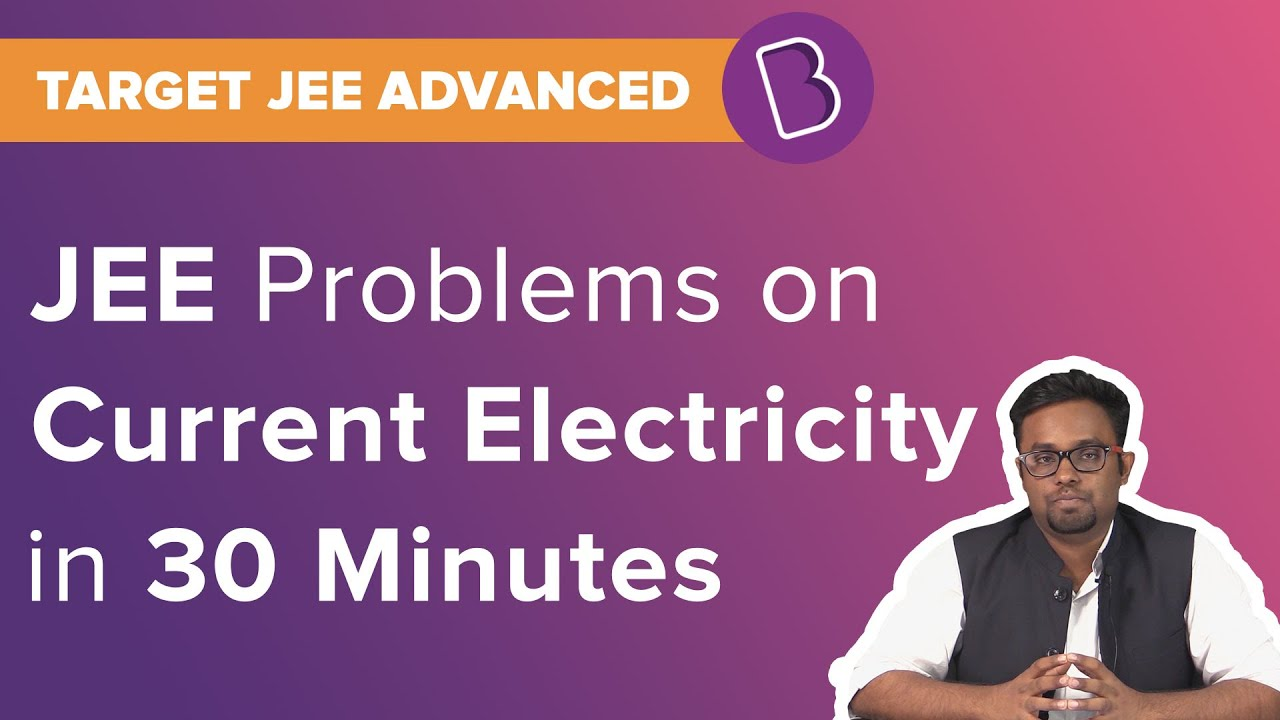Checkout JEE MAINS 2022 Question Paper Analysis : Checkout JEE MAINS 2022 Question Paper Analysis :

# Current Electricity JEE Advanced Previous Year Questions With Solutions

The branch of physics which deals with the dynamics of charges is called current electricity. The charges, in general, can be positive ions, negative ions, electrons or holes. A current is said to exist in the wire only when there is a net flow of charge through any section of the wire. The conventional direction of the current is the direction in which positive charges flow, namely from the point of higher potential to the point of lower potential.

Question 1) Two cells of emf 2E and E with internal resistance r1 and r2 respectively are connected in series to an external resistor R (see figure). The value of R, at which the potential difference across the terminals of the first cell becomes zero is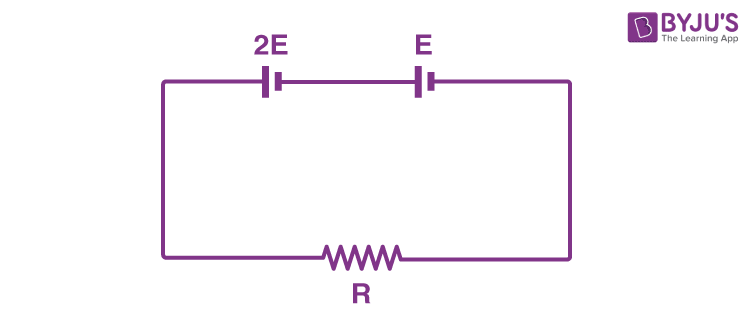(A) r1 – r2

(B) r1 + r2

(C) (r1/2) + r2

(D) (r1/2) – r2

Solution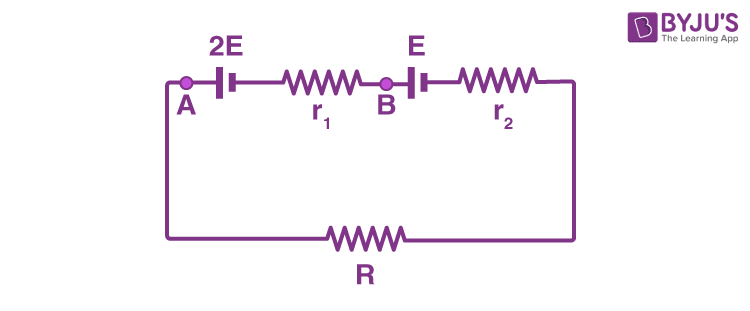$$\begin{array}{l}i = \frac{3E}{R+r_{1}+r_{2}}\end{array}$$If potential difference across terminals of first cell is zero

VA = VB

2 E = i r1

$$\begin{array}{l}2E = \frac{3E}{R+r_{1}+r_{2}}r_{1}\end{array}$$

2R + 2r1 + 2r2 = 3r1

R = (r1/2)- r2

Question 2) The four arms of a Wheatstone bridge have resistances as shown in the figure. A galvanometer of 15 resistance is connected across BD. Calculate the current through the galvanometer when a potential difference of 10 V is maintained across AC.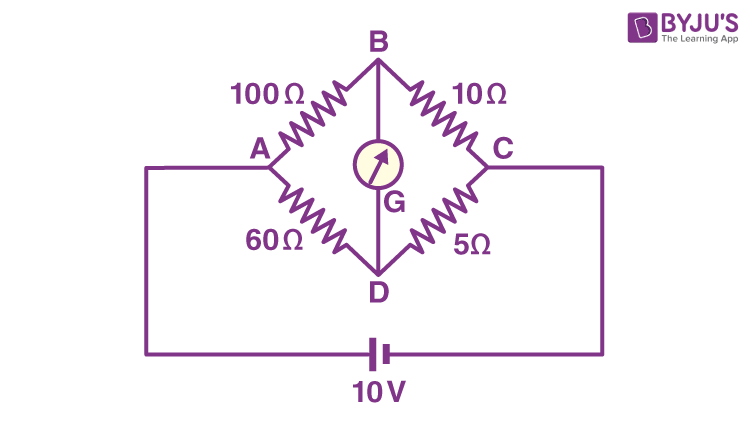(A) 4.87 mA

(B) 4.87 μA

(C) 2.44 μA

(D) 2.44 mA

Solution: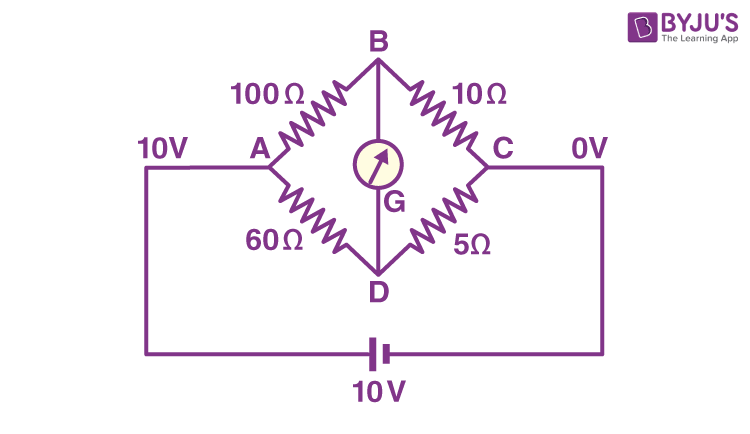Applying KCL for point B,

$$\begin{array}{l}\frac{V_{B}-10}{100}+\frac{V_{B}-V_{D}}{15}+\frac{V_{B}-0}{10}=0\end{array}$$
$$\begin{array}{l}\frac{V_{B}-10}{20}+\frac{V_{B}-V_{D}}{3}+\frac{V_{B}-0}{2}=0\end{array}$$

3VB-30+20VB-20VD+30VB=0

53VB – 20VD = 30 ____(1)

Similarly applying KCL for point D,

$$\begin{array}{l}\frac{V_{D}-10}{60}+\frac{V_{D}-V_{B}}{15}+\frac{V_{D}-0}{5}=0\end{array}$$

VD – 10 + 4VD – 4VB + 12VD = 0

– 4VB + 17VD = 10 _____(2)

after solving equation (1) & (2)

VD = 0.792 volt

VB = 0.865 volt

Then the current through the galvanometer

$$\begin{array}{l}=\frac{V_{B}-V_{D}}{R}\end{array}$$
$$\begin{array}{l}=\frac{0.865-0.792}{15}\end{array}$$

= 4.67 mA

Question 3) What happens to the inductive reactance and the current in a purely inductive circuit if the frequency is halved?

(A) Both , including reactance and current will be doubled

(B) Both, inductive reactance and current will be halved

(C) Inductive reactance will be halved and current will be doubled

(D) Inductive reactance will be doubled and current will be halved.

Solution

XL = ωL

If frequency is halved, X’L=(XL/2)

[inductive reactance is halved]

Therefore, I = V/XL

& I’ = 2V/XL=2I [current will be doubled]

Question 4) The electric field intensity produced by the radiation coming from a 100 W bulb at a distance of 3 m is E. The electric field intensity produced by the radiation coming from 60 W at the same distance is √(x/5)E. Where the value of x = ________

Solution:

I = 1/2(C∈0E2)

where E is electric field intensity at a point

E2 ∝ I

I = Power/Area

E2 ∝ (P/A)

E ∝ √P

[at the same distance, A will be the same]
$$\begin{array}{l}\frac{E’}{E}=\sqrt{\frac{60}{100}}\end{array}$$
$$\begin{array}{l}E’=\sqrt{\frac{3}{5}}E\end{array}$$

So the value of x = 3

Question 5) A 2μF capacitor C1 is first charged to a potential difference of 10V using a battery. Then the battery is removed and the capacitor is connected to an uncharged capacitor C2 of 8 μF. The charge in C2 on equilibrium conditions is ________ μC. (Round off to the Nearest Integer)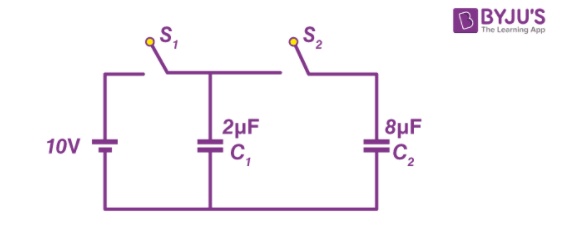Solution

After capacitor C1 is fully charged,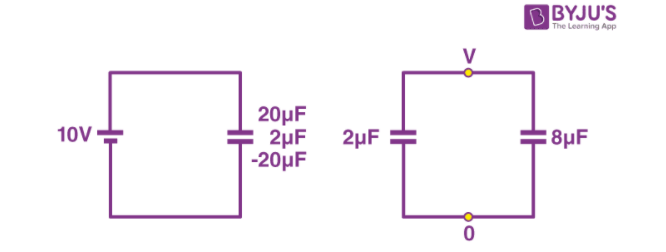When battery is removed & the capacitor is connected

At equilibrium condition, let voltage across each capacitor be V.

Then, using conservation of charge

2V + 8V = 20

10V = 20

V = 2 volt

Q = CV

Q = 8×2 = 16 μc

Question 6) The electric field in a region is given by

$$\begin{array}{l}\vec{E}=\frac{2}{3}E_{0}i+\frac{3}{5}E_{0}j\end{array}$$
with E0=4.0×103N/C. The flux of this field through a rectangular surface are 0.4 m2 parallel to Y–Z plane is ________Nm2C-1.

Solution:

From Gauss’ law

$$\begin{array}{l}\phi =\oint \vec{E}.d\vec{A}\end{array}$$

= (⅔)E0 x (0.4)

= (⅔) x 4 x 103 x 0.4

Φ = 640 Nm2 c-1

Question 7) In the given circuit find the current through 6Ω resistance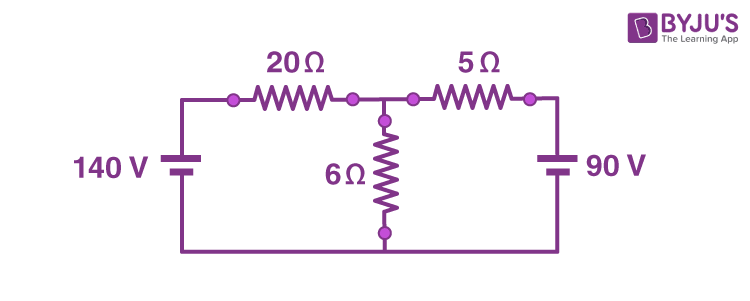(A) 10 A

(B) 7 A

(C) 25 A

(D) 30 A

Solution:

Applying Kirchoff’s law,

$$\begin{array}{l}\frac{V-140}{20}+\frac{V-0}{6}+\frac{V-90}{5}=0\end{array}$$

3(V-140)+10V+(V-90) =0

3V-420+10V+12V-1080 = 0

25V= 1500

V = 60

Current through 6Ω resistance, I = V/R = 60/6 = 10 A

Question 8) An AC circuit consists of a series combination of an inductance L 1 mH, a resistance R = 1Ω and a capacitance C. It is observed that the current leads the voltage by 45°. Find the value of capacitance ‘C’ if the angular frequency of applied AC is 200 rad/s.(A) 5.6 mF

(B) 3.92 mF

(C) 4.16 mF

(D) 5.2 mF

Solution

$$\begin{array}{l}tan \Phi =\frac{X_{c}-X_{L}}{R}=\frac{\frac{1}{\omega C}-\omega L}{R}\end{array}$$
$$\begin{array}{l}1(1)=\frac{1}{200C}-200(10^{-3})\end{array}$$
$$\begin{array}{l}\frac{1}{200C}= 1+0.2 = 1.2\end{array}$$
$$\begin{array}{l}C = \frac{1}{200\times 1.2}=0.00416 F = 4.16 mF\end{array}$$

Question 9) In a magnesium rod of area 3m2, current I = 5𝐴 is flowing angle of 600 from the axis of the rod. The resistivity of the material is 44 × 10−2 ohm x m. Find an electric field inside the rod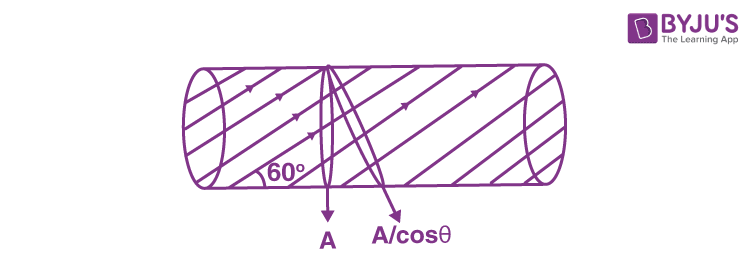(A) 0.567

(B) 0.367

(C) 0.667

(D) 0.767

Solution

$$\begin{array}{l}\frac{I}{A_{effective}}=\frac{E}{\rho }\end{array}$$
$$\begin{array}{l}E = \frac{\rho I}{A}cos 60^{0}=\frac{44\times 10^{-2}\times 5}{3\times 2}\end{array}$$

E = 0.367

Question 10) A RLC circuit is in its resonance condition. Its circuit components have value

R = 5Ω, L = 2H, C = 0.5mF, V= 250V

Then find the power in the circuit.

(A) 6 kW

(B) 10 kW

(C) 12 kW

(D) 12.5 kW